# SVM Hyperparameter Tuning using GridSearchCV | ML

• Difficulty Level : Easy
• Last Updated : 23 Aug, 2022

A Machine Learning model is defined as a mathematical model with a number of parameters that need to be learned from the data. However, there are some parameters, known as Hyperparameters and those cannot be directly learned. They are commonly chosen by humans based on some intuition or hit and trial before the actual training begins. These parameters exhibit their importance by improving the performance of the model such as its complexity or its learning rate. Models can have many hyper-parameters and finding the best combination of parameters can be treated as a search problem.
SVM also has some hyper-parameters (like what C or gamma values to use) and finding optimal hyper-parameter is a very hard task to solve. But it can be found by just trying all combinations and see what parameters work best. The main idea behind it is to create a grid of hyper-parameters and just try all of their combinations (hence, this method is called Gridsearch, But don’t worry! we don’t have to do it manually because Scikit-learn has this functionality built-in with GridSearchCV.
GridSearchCV takes a dictionary that describes the parameters that could be tried on a model to train it. The grid of parameters is defined as a dictionary, where the keys are the parameters and the values are the settings to be tested.
This article demonstrates how to use the GridSearchCV searching method to find optimal hyper-parameters and hence improve the accuracy/prediction results

### Import necessary libraries and get the Data:

We’ll use the built-in breast cancer dataset from Scikit Learn. We can get with the load function:

## Python3

 `import` `pandas as pd``import` `numpy as np``from` `sklearn.metrics ``import` `classification_report, confusion_matrix``from` `sklearn.datasets ``import` `load_breast_cancer``from` `sklearn.svm ``import` `SVC`` ` `cancer ``=` `load_breast_cancer()`` ` `# The data set is presented in a dictionary form:``print``(cancer.keys())`

`dict_keys(['data', 'target', 'target_names', 'DESCR', 'feature_names', 'filename'])`

Now we will extract all features into the new data frame and our target features into separate data frames.

## Python3

 `df_feat ``=` `pd.DataFrame(cancer[``'data'``],``                       ``columns ``=` `cancer[``'feature_names'``])`` ` `# cancer column is our target``df_target ``=` `pd.DataFrame(cancer[``'target'``], ``                     ``columns ``=``[``'Cancer'``])`` ` `print``(``"Feature Variables: "``)``print``(df_feat.info())`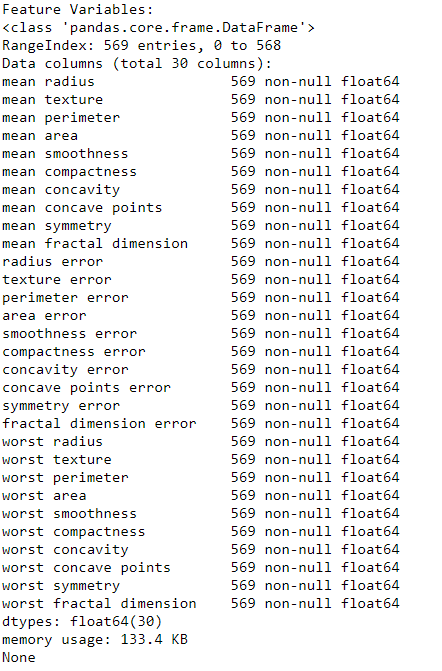## Python3

 `print``(``"Dataframe looks like : "``)``print``(df_feat.head())`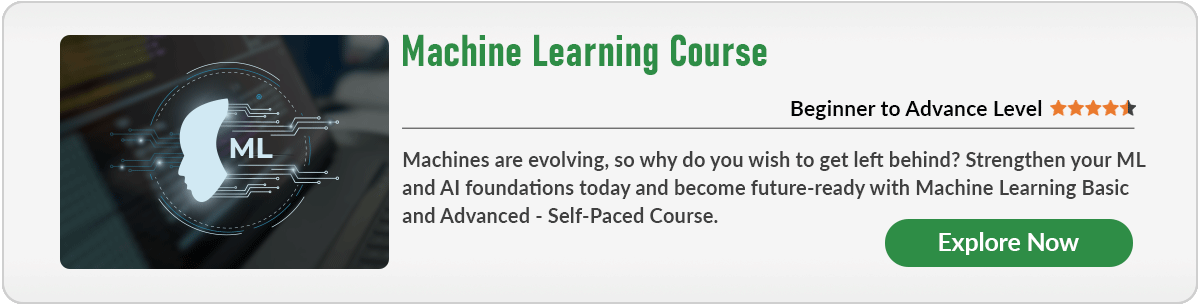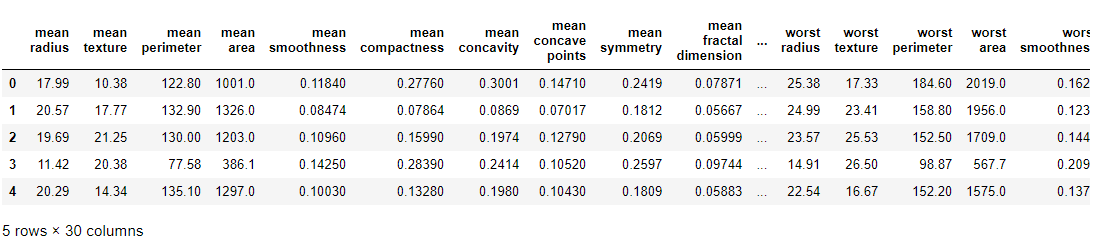### Train Test Split

Now we will split our data into train and test set with a 70: 30 ratio

## Python3

 `from` `sklearn.model_selection ``import` `train_test_split`` ` `X_train, X_test, y_train, y_test ``=` `train_test_split(``                        ``df_feat, np.ravel(df_target),``                ``test_size ``=` `0.30``, random_state ``=` `101``)`

### Train the Support Vector Classifier without Hyper-parameter Tuning –

First, we will train our model by calling the standard SVC() function without doing Hyperparameter Tuning and see its classification and confusion matrix.

## Python3

 `# train the model on train set``model ``=` `SVC()``model.fit(X_train, y_train)`` ` `# print prediction results``predictions ``=` `model.predict(X_test)``print``(classification_report(y_test, predictions))`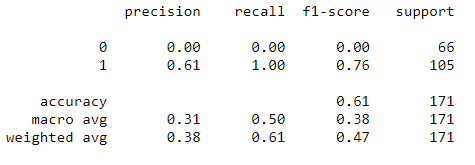We got 61 % accuracy but did you notice something strange?
Notice that recall and precision for class 0 are always 0. It means that the classifier is always classifying everything into a single class i.e class 1! This means our model needs to have its parameters tuned.
Here is when the usefulness of GridSearch comes into the picture. We can search for parameters using GridSearch!

### Use GridsearchCV

One of the great things about GridSearchCV is that it is a meta-estimator. It takes an estimator like SVC and creates a new estimator, that behaves exactly the same – in this case, like a classifier. You should add refit=True and choose verbose to whatever number you want, the higher the number, the more verbose (verbose just means the text output describing the process).

## Python3

 `from` `sklearn.model_selection ``import` `GridSearchCV`` ` `# defining parameter range``param_grid ``=` `{``'C'``: [``0.1``, ``1``, ``10``, ``100``, ``1000``], ``              ``'gamma'``: [``1``, ``0.1``, ``0.01``, ``0.001``, ``0.0001``],``              ``'kernel'``: [``'rbf'``]} `` ` `grid ``=` `GridSearchCV(SVC(), param_grid, refit ``=` `True``, verbose ``=` `3``)`` ` `# fitting the model for grid search``grid.fit(X_train, y_train)`

What fit does is a bit more involved than usual. First, it runs the same loop with cross-validation, to find the best parameter combination. Once it has the best combination, it runs fit again on all data passed to fit (without cross-validation), to build a single new model using the best parameter setting.
You can inspect the best parameters found by GridSearchCV in the best_params_ attribute, and the best estimator in the best_estimator_ attribute:

## Python3

 `# print best parameter after tuning``print``(grid.best_params_)`` ` `# print how our model looks after hyper-parameter tuning``print``(grid.best_estimator_)`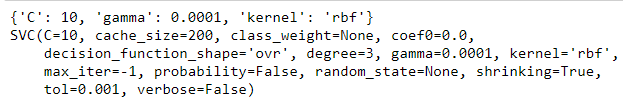Then you can re-run predictions and see a classification report on this grid object just like you would with a normal model.

## Python3

 `grid_predictions ``=` `grid.predict(X_test)`` ` `# print classification report``print``(classification_report(y_test, grid_predictions))`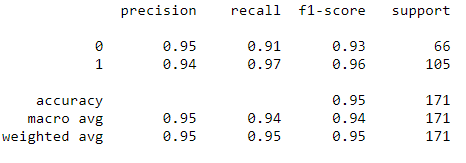We have got almost 95 % prediction result.

My Personal Notes arrow_drop_up